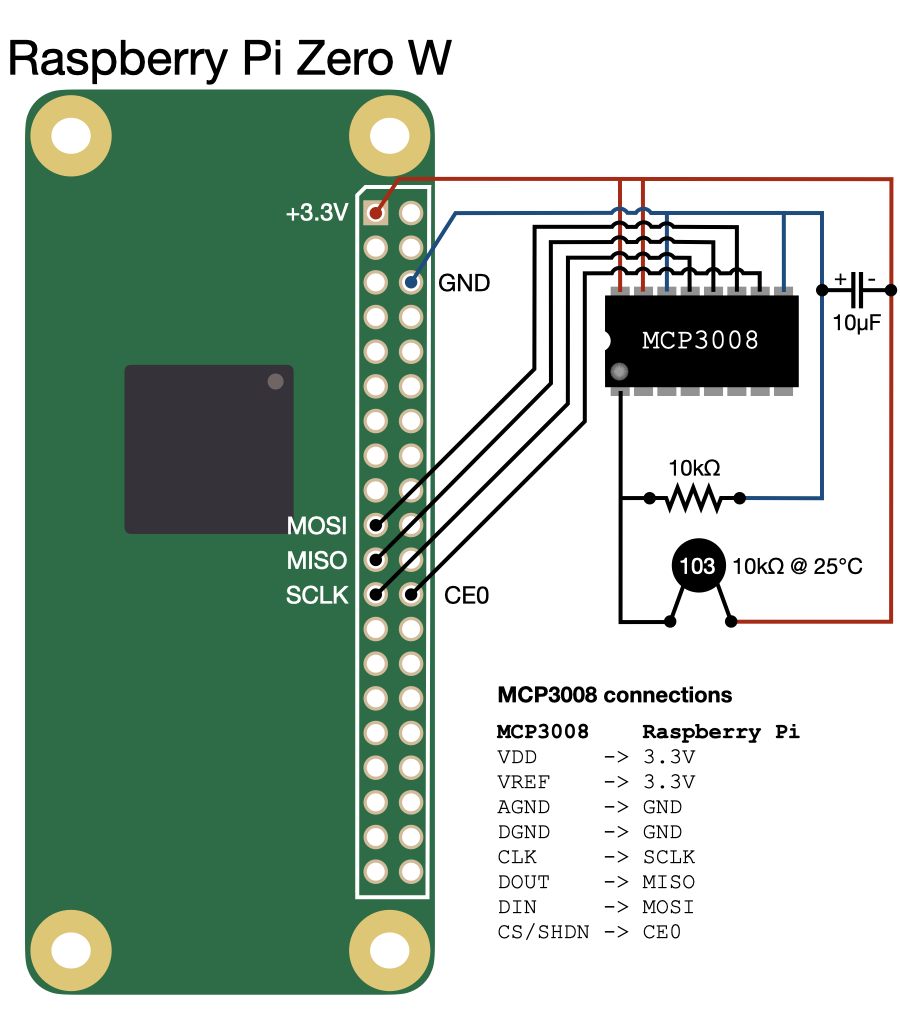Temperature

# Temperature

This example shows how to use BaremetalPi.jl together with a AD converter MCP3008 and a 103 thermistor to read the ambient temperature. The connections between the components are shown in the following figure:

Warning

We are using the Raspberry Pi Zero W as an example. You must modify it according to your model.The MCP3008 is a 8-channel 10-bit analog to digital converted that communicates using the SPI interface. Thus, the first thing is to make sure that SPI is enabled in your Linux distribution so that the devices `/dev/spidevX.Y` exists (`X` and `Y` are integers related to the SPI numbering). Please, check the manual of your distribution for more information.

After the connection, we need to start the SPI interface in BaremetalPi.jl. Here, we consider that the device in which the MCP3008 was connected is called `/dev/spidev0.0`.

``````using BaremetalPi

init_spi("/dev/spidev0.0", max_speed_hz = 1000)``````

Notice that we limit the sampling speed to 1000 Hz to improve the signal-to-noise ratio of the AD conversion as per MCP3008 datasheet.

To acquire a new measurement, we need to perform a full-duplex SPI transfer. Three bytes must be sent to the device: `0x01 0x80 0x00`, which asks for a new measurement of the channel 0. For more information about how MCP3008 works, please, see the datasheet. This full-duplex transfer can be accomplished using BaremetalPi.jl as follows:

``````tx_buf = [0x01, 0x80, 0x00]
rx_buf = zeros(UInt8, 3)

ret = spi_transfer!(1, tx_buf, rx_buf)``````

`ret` must be 3 indicating that the device returned 3 bytes. The 10-bit AD measurement is then stored in `rx_buf[2:3]`. The conversion to voltage is performed as follows:

``V = ( (UInt16(rx_buf & 3) << 8) + rx_buf )*3.3/1024``

Now, we need to obtain the resistance of the thermistor using the equation of the voltage divider:

``````R_div = 10_000         # ............. Resistor value at the voltage divider [Ω]
th_R = R_div*(3.3/V - 1)``````

Finally, using the relationship between resistance and temperature for the selected thermistor, we can get a measurement of the ambient temperature:

``````th_β  = 3380           # ................ Beta coefficient of the thermistor [K]
th_R₀ = 10_000         # ............. Reference thermistor resistance at T₀ [Ω]
th_T₀ = 25             # ....... Reference temperature T₀ of the thermistor [°C]

T = 1/( log(th_R / th_R₀)/th_β + 1/(th_T₀ + 273.15) ) - 273.15``````

The following code prints the temperature every 5s:

``````using Dates
using Printf
using BaremetalPi

################################################################################
#                                Configuration
################################################################################

const R_div = 10_000   # ............. Resistor value at the voltage divider [Ω]
const th_β  = 3380     # ................ Beta coefficient of the thermistor [K]
const th_R₀ = 10_000   # ............. Reference thermistor resistance at T₀ [Ω]
const th_T₀ = 25       # ....... Reference temperature T₀ of the thermistor [°C]

################################################################################
#                                  Functions
################################################################################

function acquire_temperature()
# ==========================================================================

tx_buf = [0x01, 0x80, 0x00]
rx_buf = zeros(UInt8, 3)
V      = 0

ret = spi_transfer!(1, tx_buf, rx_buf)

if ret != 3
@warn("The data received from MCP3008 does not have 3 bytes.")
return NaN
end

V = ( (UInt16(rx_buf & 3) << 8) + rx_buf )*3.3/1024

if V == 0
@warn("The MCP3008 measured 0V.")
return NaN
end

if V > 3.25
@warn("The MCP3008 measured a too high voltage (> 3.25V).")
return NaN
end

# Convert the measurement to temperature
# ==========================================================================

th_R = R_div*(3.3/V - 1)
T    = 1/( log(th_R / th_R₀)/th_β + 1/(th_T₀ + 273.15) ) - 273.15

return T
end

function run()
# Let's sample at 1kHz to improve the accuracy of MCP3008.
init_spi("/dev/spidev0.0", max_speed_hz = 1000)

while true
T = acquire_temperature()

if !isnan(T)
@printf("%-30s %3.2f\n", string(now()), T)
else
println("[ERROR] Problem when acquiring AD measurement.")
end
sleep(5)
end
end

run()``````

Result:

``````2020-06-14T19:36:40.564        22.65
2020-06-14T19:36:46.235        22.65
2020-06-14T19:36:51.264        22.65
2020-06-14T19:36:56.286        22.65
2020-06-14T19:37:01.32         22.65
2020-06-14T19:37:06.354        22.65
2020-06-14T19:37:11.381        22.65
2020-06-14T19:37:16.415        22.65
2020-06-14T19:37:21.443        22.65
2020-06-14T19:37:26.465        23.57   -> I placed my finger on the thermistor.
2020-06-14T19:37:31.497        23.57
2020-06-14T19:37:36.525        23.16
2020-06-14T19:37:41.547        22.86
2020-06-14T19:37:46.581        22.76``````
Info

To improve the accuracy, measure the resistance of the voltage divider resistor and update the constant `R_div`. Furthermore, check the β of your thermistor and update the value in the constant `th_β`.